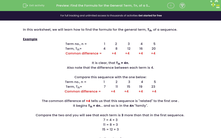# Find the Formula for the General Term, Tn, of a Sequence

In this worksheet, studends find the formula for the general term, Tn, of a sequence.Key stage:  KS 3

Curriculum topic:   Algebra

Curriculum subtopic:   Understand Expressions, Equations, Inequalities, Terms and Factors

Difficulty level:#### Worksheet Overview

In this worksheet, we will learn how to find the formula for the general term, Tn, of a sequence.

Example

Term no., n =                   1           2            3            4            5
Term, Tn=                        4           8           12          16          20
Common difference =           +4          +4          +4         +4

It is clear, that Tn = 4n.

Also note that the difference between each term is 4.

Compare this sequence with the one below:

Term no., n =                   1            2            3            4            5
Term, Tn=                        7           11          15          19          23
Common difference =           +4          +4           +4          +4

The common difference of +4 tells us that this sequence is "related" to the first one .

It begins Tn = 4n... and so is in the 4n "family".

Compare the two and you will see that each term is 3 more than that in the first sequence.

7 = 4 + 3
11 = 8 + 3
15 = 12 + 3

So Tn = 4n + 3

### What is EdPlace?

We're your National Curriculum aligned online education content provider helping each child succeed in English, maths and science from year 1 to GCSE. With an EdPlace account you’ll be able to track and measure progress, helping each child achieve their best. We build confidence and attainment by personalising each child’s learning at a level that suits them.

Get started